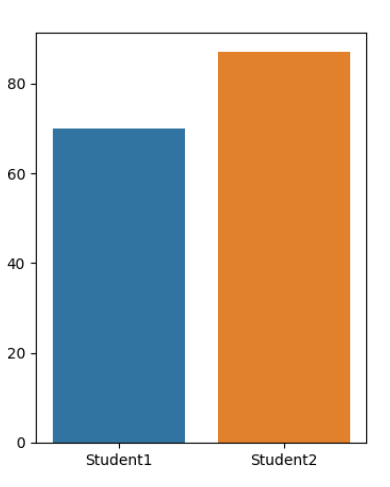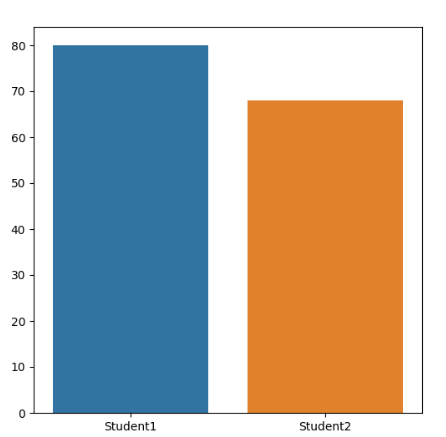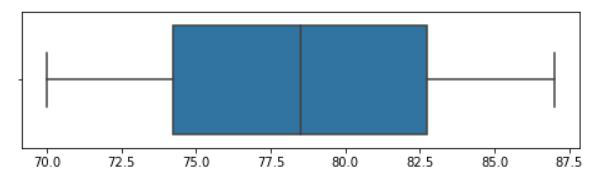# How to set a Seaborn chart figure size?

• Last Updated : 24 Feb, 2021

Seaborn is a Python data visualization library based on matplotlib. It is used to draw attractive and informative statistical graphics. To adjust the figure size of the seaborn plot we will use the subplots function of matplotlib.pyplot.

matplotlib.pyplot.subplots() Create a figure and a set of subplots. It has a parameter called figsize which takes a tuple as an argument that contains the height and the width of the plot. It returns the figure and the array of axes.

While calling the seaborn plot we will set the ax parameter equal to the array of axes that was returned by matplotlib.pyplot.subplots after setting the dimensions of the desired plot.

Example 1: We will consider two students and plot their marks in a bar plot, and we will set the plot of size ( 4,5 ).

## Python3

 `# Importing libraries ` `import` `seaborn as sns ` `import` `matplotlib.pyplot as plt ` ` `  `# Setting the data ` `x ``=` `[``"Student1"``, ``"Student2"``] ` `y ``=` `[``70``, ``87``] ` ` `  `# setting the dimensions of the plot ` `fig, ax ``=` `plt.subplots(figsize``=``(``4``, ``5``)) ` ` `  `# drawing the plot ` `sns.barplot(x, y, ax``=``ax) ` `plt.show() `

Output:fig size ( 6,4 )

Example 2: We will draw a plot of size (6, 6).

## Python3

 `# Importing libraries ` `import` `seaborn as sns ` `import` `matplotlib.pyplot as plt ` ` `  `# Setting the data ` `x ``=` `[``"Student1"``, ``"Student2"``] ` `y ``=` `[``80``, ``68``] ` ` `  `# setting the dimensions of the plot ` `fig, ax ``=` `plt.subplots(figsize``=``(``6``, ``6``)) ` ` `  `# drawing the plot ` `sns.barplot(x, y, ax``=``ax) ` `plt.show() `fig size ( 6,6 )

Example 3: In this example, We will create boxplot and set the size of a chart with figsize.

## Python3

 `# Importing libraries ` `import` `seaborn as sns ` `import` `matplotlib.pyplot as plt ` ` `  `# Setting the data ` `x ``=` `[``"Student1"``, ``"Student2"``] ` `y ``=` `[``70``, ``87``] ` ` `  `# setting the dimensions of the plot ` `fig, ax ``=` `plt.subplots(figsize``=``(``40``, ``5``)) ` ` `  `# drawing the plot ` `sns.boxplot(x ``=` `y) ` `plt.show() `

Output:My Personal Notes arrow_drop_up
Recommended Articles
Page :